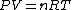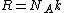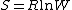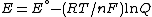# gas constant

(redirected from gas constants)
Also found in: Dictionary, Thesaurus, Medical.

## Gas constant

The universal constant R

(1)that appears in the ideal gas law, Eq. (1), where P is the pressure, V the volume, n the amount of substance, and T the thermodynamic (absolute) temperature. The gas constant is universal in that it applies to all gases, providing they are behaving ideally (in the limit of zero pressure). The gas constant is related to the more fundamental Boltzmann constant, k, by
(2)Eq. (2), where NA is the Avogadro constant (the number of entities per mole). The best modern value in SI units is R = 8.314 472 (15) J/K &cdot; mol, where the number in parentheses represents the uncertainty in the last two digits. See Boltzmann constant

According to the equipartition principle, at a temperature T, the average molar energy of each quadratic term in the expression for the energy is (1/2)RT; as a consequence, the translational contribution to the molar heat capacity of a gas at constant volume is (3/2)R; the rotational contribution of a linear molecule is R. See Kinetic theory of matter

Largely because R is related to the Boltzmann constant, it appears in a wide variety of contexts, including properties unrelated to gases. Thus, it occurs in Boltzmann's formula for the molar entropy of any substance, Eq. (3),

(3)where W is the number of arrangements of the system that are consistent with the same energy; and in the Nernst equation for the potential of an electrochemical cell, Eq. (4),
(4)where E° is a standard potential, F is the Faraday constant, and Q is a function of the composition of the cell. The gas constant also appears in the Boltzmann distribution for the population of energy levels when the energy of a level is expressed as a molar quantity. See Boltzmann statistics, Entropy

McGraw-Hill Concise Encyclopedia of Physics. © 2002 by The McGraw-Hill Companies, Inc.

## gas constant

[′gas ‚kän·stənt]
(thermodynamics)
The constant of proportionality appearing in the equation of state of an ideal gas, equal to the pressure of the gas times its molar volume divided by its temperature. Also known as gas-law constant; universal gas constant.
McGraw-Hill Dictionary of Scientific & Technical Terms, 6E, Copyright © 2003 by The McGraw-Hill Companies, Inc.
Site: Follow: Share:
Open / Close